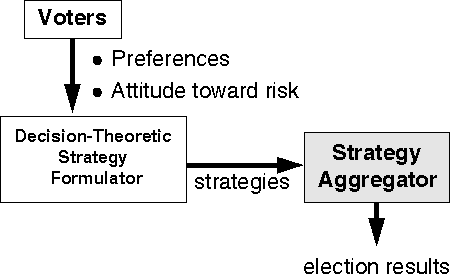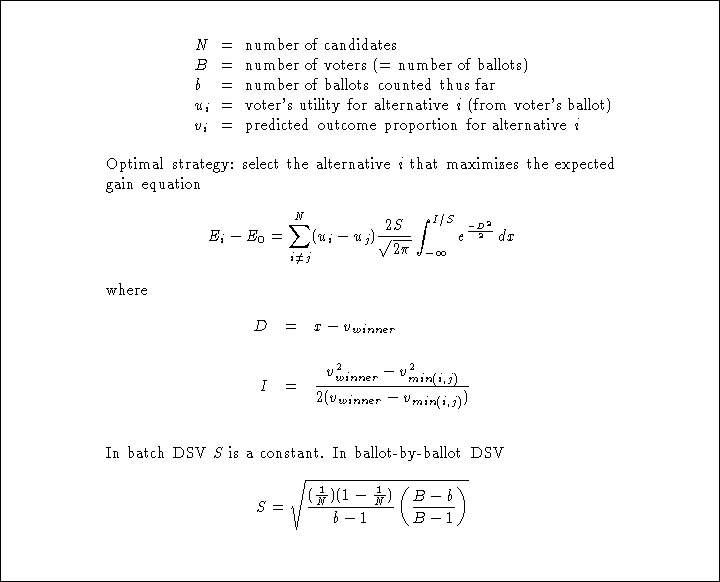Next: Evaluation of Declared Strategy Up: Rational Strategy Formulation Previous: Tie-Breaking Rules

# The Decision-Theoretic Strategy Formulator

In this chapter we have seen how the expected-utility model can be used to produce optimal strategies based on a voter's preferences and predictions about the outcome of an election. A voter's preferences may be represented as utility values on a finite scale, say from 0 to 10. In addition, voters who are indifferent between alternatives must also supply tie-breaking rules. Depending on the pivot-probability method used, we might also ask voters to supply a measure of their attitude towards risk. Given this information, a computer can calculate the optimal decision-theoretic strategy for each voter and submit it to the strategy aggregator, as shown in Figure. Thus, voters need not understand the process of formulating an expected-utility maximization function to use this system.Figure: A model of declared-strategy voting with decision-theoretic strategy formulator

A strategy formulator that uses our 2-D Gaussian method for calculating pivot probabilities formulates an optimal strategy for an N-alternative plurality-rule election as shown in Figure.Figure: Summary of formulas for optimal plurality-rule strategy with 2-D Gaussian pivot probabilities

Note that we ask voters to submit their sincere utility values for all candidates. Our system calculates the optimal decision-theoretic strategy based on these values, enabling voters to vote strategically while simultaneously revealing their sincere preferences. Thus it is generally advantageous for voters to submit their sincere utility values. However, as we discuss later, occasionally voters who have the necessary knowledge to formulate sophisticated strategies might not find it to their advantage to reveal their sincere utility values (and will act accordingly).

lorrie@acm.org